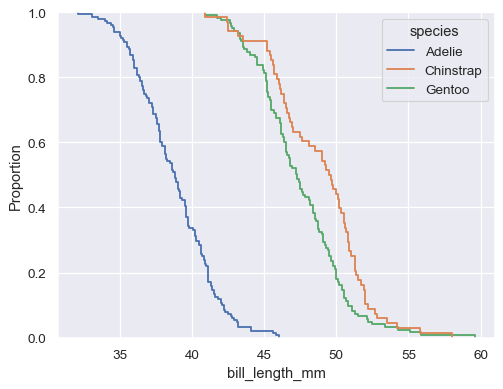# seaborn.ecdfplot¶

`seaborn.``ecdfplot`(data=None, *, x=None, y=None, hue=None, weights=None, stat='proportion', complementary=False, palette=None, hue_order=None, hue_norm=None, log_scale=None, legend=True, ax=None, **kwargs)

Plot empirical cumulative distribution functions.

An ECDF represents the proportion or count of observations falling below each unique value in a dataset. Compared to a histogram or density plot, it has the advantage that each observation is visualized directly, meaning that there are no binning or smoothing parameters that need to be adjusted. It also aids direct comparisons between multiple distributions. A downside is that the relationship between the appearance of the plot and the basic properties of the distribution (such as its central tendency, variance, and the presence of any bimodality) may not be as intuitive.

Parameters
data`pandas.DataFrame`, `numpy.ndarray`, mapping, or sequence

Input data structure. Either a long-form collection of vectors that can be assigned to named variables or a wide-form dataset that will be internally reshaped.

x, yvectors or keys in `data`

Variables that specify positions on the x and y axes.

huevector or key in `data`

Semantic variable that is mapped to determine the color of plot elements.

stat{{“proportion”, “count”}}

Distribution statistic to compute.

complementarybool

If True, use the complementary CDF (1 - CDF)

palettestring, list, dict, or `matplotlib.colors.Colormap`

Method for choosing the colors to use when mapping the `hue` semantic. String values are passed to `color_palette()`. List or dict values imply categorical mapping, while a colormap object implies numeric mapping.

hue_ordervector of strings

Specify the order of processing and plotting for categorical levels of the `hue` semantic.

hue_norm

Either a pair of values that set the normalization range in data units or an object that will map from data units into a [0, 1] interval. Usage implies numeric mapping.

log_scalebool or number, or pair of bools or numbers

Set a log scale on the data axis (or axes, with bivariate data) with the given base (default 10), and evaluate the KDE in log space.

legendbool

If False, suppress the legend for semantic variables.

ax`matplotlib.axes.Axes`

Pre-existing axes for the plot. Otherwise, call `matplotlib.pyplot.gca()` internally.

kwargs

Other keyword arguments are passed to `matplotlib.axes.Axes.plot()`.

Returns
`matplotlib.axes.Axes`

The matplotlib axes containing the plot.

`displot`

Figure-level interface to distribution plot functions.

`histplot`

Plot a histogram of binned counts with optional normalization or smoothing.

`kdeplot`

Plot univariate or bivariate distributions using kernel density estimation.

`rugplot`

Plot a tick at each observation value along the x and/or y axes.

Examples

Plot a univariate distribution along the x axis:

```penguins = sns.load_dataset("penguins")
sns.ecdfplot(data=penguins, x="flipper_length_mm")
```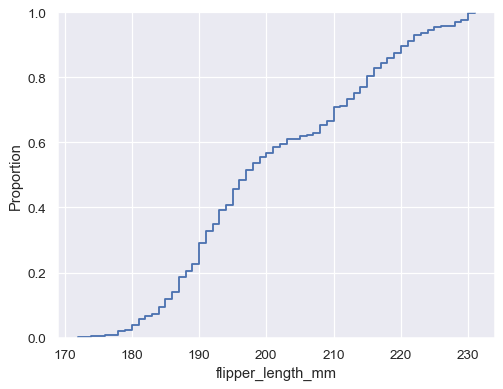Flip the plot by assigning the data variable to the y axis:

```sns.ecdfplot(data=penguins, y="flipper_length_mm")
```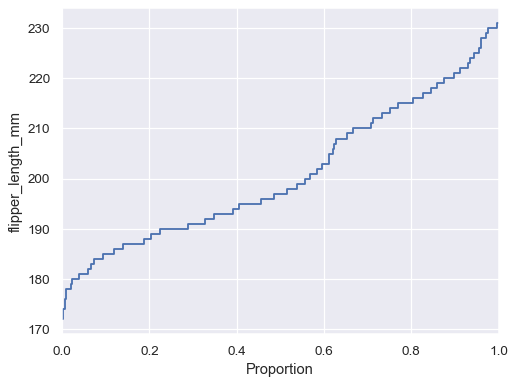If neither `x` nor `y` is assigned, the dataset is treated as wide-form, and a histogram is drawn for each numeric column:

```sns.ecdfplot(data=penguins.filter(like="bill_", axis="columns"))
```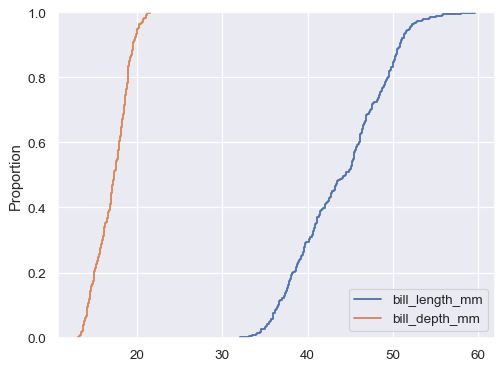You can also draw multiple histograms from a long-form dataset with hue mapping:

```sns.ecdfplot(data=penguins, x="bill_length_mm", hue="species")
```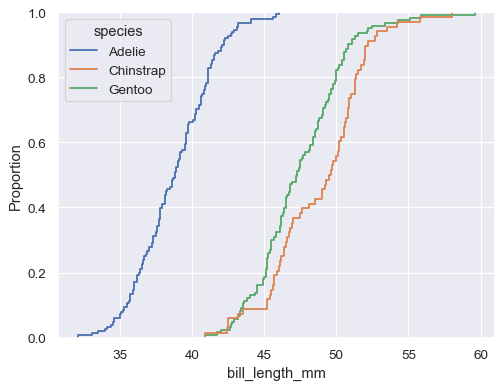The default distribution statistic is normalized to show a proportion, but you can show absolute counts instead:

```sns.ecdfplot(data=penguins, x="bill_length_mm", hue="species", stat="count")
```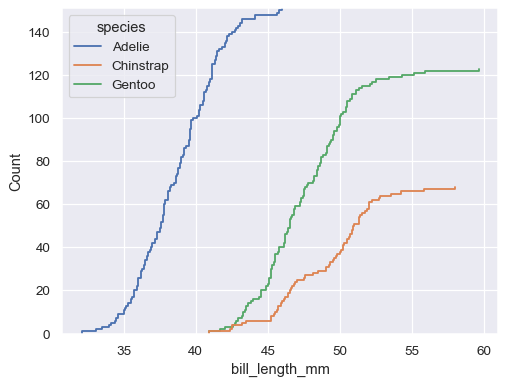It’s also possible to plot the empirical complementary CDF (1 - CDF):

```sns.ecdfplot(data=penguins, x="bill_length_mm", hue="species", complementary=True)
```